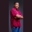Related Tags

range
contains
swift
communitycreator

# How to check if a range contains a particular valueTheodore Kelechukwu Onyejiaku

### Overview

With the contains method, we can check if a range in Swift contains a particular value. If it does, a true Boolean value is returned. Otherwise, a false will be returned.

### Syntax

range.contains(value)


### Parameters

value: This is the value we check to see if the range instance range contains it.

### Return value

The value returned is a Boolean. If the value value is contained in the range instance range, then true is returned. Otherwise, false is returned.

### Code example

// create a range
let range1 = 0 ..< 5 // 0 to 4
// print each value in the range
print("Printing range elements: ")
for value in range1 {
print(value)
}
// check if range contains some value
print("checking if range contains some value")
print("1:", range1.contains(1))   // true
print("5:", range1.contains(5))   // false
print("10:", range1.contains(10)) // false
print("3:", range1.contains(3))   // true
Checking if a particular value is present in a range

### Explanation

• Line 2: We create a range called range1.
• Line 5: Using the for-in loop, we print all the values that are present in the range.
• Lines 10 to 13: We check some values to see if they were present in the range that we created using the contains() method. We then print the results to the console.

RELATED TAGS

range
contains
swift
communitycreator

CONTRIBUTORTheodore Kelechukwu Onyejiaku
RELATED COURSES

View all Courses

Keep Exploring

Learn in-demand tech skills in half the time